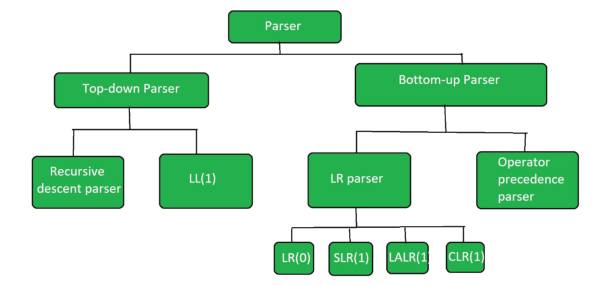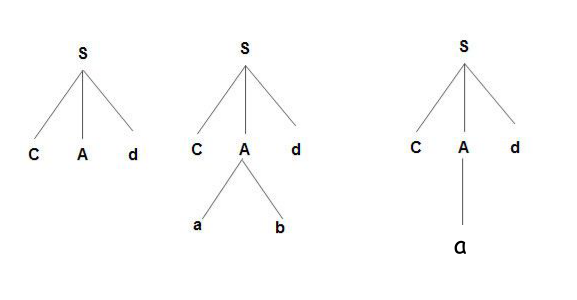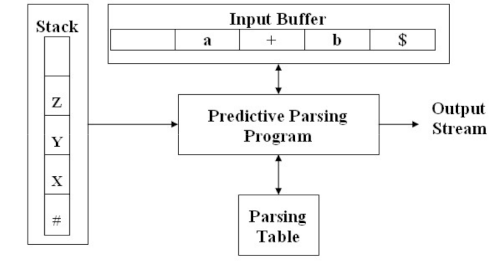×

Search anything:

# Parsing in Compiler Design

#### Compiler Design Software EngineeringGet this book -> Problems on Array: For Interviews and Competitive Programming

In this article, we discuss the different types of Parsing done by compilers such as Top-down parsing, Non-recursive predictive parsing and much more.

1. Introduction to Parsing
2. Top-down parsing (LL)
• Recursive descent
• Non-Recursive predictive parser (LL)
3. Bottom Up parsing (LR Parsing)
• Shift reduce parsing (LR)
• LR(0) Parsing
• SLR(1) Parsing
• LALR(1) Parsing
• CLR(1) Parsing
• Operator precedence parsing

## Introduction to Parsing

Parsing or (syntax analysis) is the process of analyzing text containing a sequence of tokens to determine its grammatical structure with respect to grammar.

### Categories of parsing

1. Universal parsing.
2. Top-down parsing.
3. bottom-up parsing.

We shall be discussing the two commonly used parsing techniques, that is top-down and bottom-up parsing.The different types of Parsing techniques in Compiler are:

• Top-down parsing (LL)
• Recursive descent
• Non-Recursive predictive parser (LL)
• Bottom Up parsing (LR Parsing)
• Shift reduce parsing (LR)
• LR(0) Parsing
• SLR(1) Parsing
• LALR(1) Parsing
• CLR(1) Parsing
• Operator precedence parsing

# 1. Top-down parsing (LL)

In this type of parsing the parse tree constructed for the input string starts from the root node and creates the nodes of the parse tree in a pre-order manner.
It is done by the left most derivation for an input string.

'L' stands for left - right scanning of the input, the other 'L' stands for left most derivation and only one non-terminal expanded at each step.

The parse tree is constructed by the parser from the start symbol and the start symbol is transformed into input.

Top-down parsing is divided into the following sub-categories;

1. Recursive descent.
2. Non-recursive predictive parsing.

## a.) Recursive descent

It is one of the simplest parsing techniques used in practice. It constructs the parse tree in a top-down manner.
The basic idea is to associate each non-terminal with a procedure that reads a sequence of input characters that can be generated by the corresponding non-terminal and return a pointer to the root of the parse tree for the non-terminal.
These procedures are recursive in nature.
A non-terminal of the current derivation step is expanded using the production rule in the given grammar.
If an expansion doesn't give the desired result the parser will drop the current production and applies another production corresponding to the same non-terminal symbol (backtracking).
The process is repeated until the required result is obtained.

Example;
Consider the grammar:
A -> ab | a#### Limitations

• Slow due to backtracking.
• Possibility of infinite loops, i.e when a grammar with left recursive production is given. that means that left recursion must be eliminated.

Recursive descent can also be done without backtracking, whereby each non-terminal is expanded by its correct alternative in the first selection.
Note that, when the correct alternative is not chosen, it will result in a syntactic error.

• Backtracking is eliminated.

#### Limitations

• Parser requires grammar without common prefixes for alternatives to make selection of the correct alternative easier.

### b.) Non-Recursive predictive parser (LL)

It is an implementation of the predictive parser that solves the problem of determining the production to be applied for a non-terminal by implementing an implicit stack and parsing table.

It looks up the production to be applied in a parsing table constructed from a certain grammar.

In case more than one production corresponds to the same non-terminal, it will attempt to predict the appropriate production to expand the non-terminal at the current derivation step.

Therefore for the construction an predictive parser we must know;

• Given the current input symbol α and the non-terminal to be expanded, which alternatives of production A -> α1 | α2 | α3 | --- | αn is an unique alternative that derives a string beginning with α.

E.g;
Given the productions:
stmt -> if expr than stmt else stmt
| while stmt than stmt
| begin stmt_list end

Then the keywords if, while, begin, tell us which alternative could possible succeed if we are to find a statement.

#### Model of non-recursive predictive parser.

Input buffer.
Contains strings to be parsed followed by a \$ symbol to indicate the end of input string.

Stack.
Contains a sequence of grammar symbols (terminal and non-terminal) with # or \$ indicating the bottom of the stack.

Parse Table.
A 2D array M[A, a] where A is a non-terminal and a is terminal of symbol \$.#### Functions for Non-recursive Predictive Parser.

Let X be the symbol at the top of the stack and a the current symbol
If X = a = \$, the parser halts and announces the completion of a successful parsing.

POP.
If X = a is not equal to \$, X is popped from the stack and the parser advances the input pointer to the next input symbol.

Apply.
If X is non-terminal it is popped from the stack.
The parser consults the parsing table M[A, a].
The entry will either be an X-production of the grammar or an error entry.
If, for example, M[X, a] = {X -> UVW}, the parser will replace X on top of the stack with WVU, with U on top of the stack.

Rejects.
If M[A, a] = error, an error recovery routing will be called.

Accepts.
If current input is \$, that is, a = \$ and top of stack is \$, that is, X = \$, the parser declares the input string valid and outputs the structure of the parser as its output.

Construction of a non-recursive predictive parser is aided by the following two functions, FIRST and FOLLOW, which allow filling in entries of a parsing table for grammar G when possible.
We need FIRST and FOLLOW sets for a given grammar so that the parser can properly apply the needed rule at the correct position.

FIRST
If the compiler would have come to know in advance, the "first character of the string produced when a production rule is applied" and compare it to the current character or token in the input string, then it can wisely take decision on which production rule to apply.

Example.
Given the grammar
A -> bc | a

If the compiler knew after reading the character 'c' and applying S->cAd, next character would be 'a' then it would have ignored the production rule A->bc(because 'b' is the first character of the string produced by this production and not 'a') and directly used the production rule A->a because 'a' is the first character of the string produced by this production rule and it is the same as the current character of the input string which is also 'a'.

FOLLOW
FOllOW can make a non-terminal vanish if needed to generate the string from the parse tree.
Consider the grammar below
A -> aBb
B- > c | ∈
Suppose the input string is "ab".

The parser applies the first rule A -> aBb because the first character in the input is a,
It checks for the second character of the input string which is b and the non-terminal to derive B.
It cant get any string derivable from B that contains b as its first character.
But then the grammar contains a production rule B -> ∈ which when applied, B will vanish and the parser will get the input "ab".
The parser can only apply it when it knows that the following character after B in the production rule is same as the current character in the input.
In RHS of A->aBa, b follows non-terminal B i.e (FOLLOW(B) = {B}) and the current input character read is also b therefore the parser will apply this rule and it will be able to get the string "ab" from the given grammar.

## 2. Bottom Up parsing (LR Parsing)

LR -> L stands for left-to-right scanning of the input and R is for right most derivation and only one non-terminal expanded at each step.

Parsing starts at the input symbol and the parse tree is constructed up to the start symbol

### a.) Shift reduce parsing (LR)

This is the process of reducing a string to the start symbol of a grammar.
It uses a stack to hold the grammar and an input tape to hold the string.

String -----reduced to----> the starting symbol.

It performs two actions, Shift and Reduce.

#### Model of shift reduce parser.

Input Buffer.
Stores the input string.

Stack.
Used for storage and access of the production rules.

#### Operations.

Shift.
The current symbol of the input string is pushed onto the stack.

Reduce.
Replaces the symbol on the right side of production with the symbol on the left side of the concerned production.
For this a parser must know the right end of the handle at the top of the stack.
Then the left end of the handle within the stack is located and the non-terminal to replace the handle is decided.
The symbols will be replaced by non-terminals.
The symbol is the right side of the production
Non-terminal is the left side of the production.

Accept.
A parsing action is called accept when the start symbol is present in the stack and the input buffer is empty therefore which means successful parsing.
The parser will announces successful completion of parsing.

Error.
Whereby the parser can not perform shift, reduce or accept actions due to a syntax error.
An error recovery routine is called.

Limitations.

• Shift-reduce conflicts -> Whereby for every shift-reduce for context free grammar, a point is reached where the parser cannot decide whether to shift or reduce.
• Reduce-reduce conflicts -> Whereby the parser cannot decide which of the several reductions to make.

### b.) LR(0) Parsing

Also know as LR(k) parsing where
"L" stands for left-to-right scanning of the input.
"R" stands for the construction of the right most derivation in reverse.
"K" stands for the number of input symbols of the look ahead used to make number of parsing decisions.
This is non-recursive shift-reduce, bottom-up parsing.
It is used to parse a large class of grammars therefore making it the most efficient syntax analysis technique.
The sub categories of LR parsing are;

• SLR(1) -> Simple LR Parsing.
• LALR(1) -> Look-Ahead LR Parsing.
• CLR(1) -> Canonical Look-Ahead LR Parsing.

We shall give in short summaries of the above and links at the reference section for further reading.

#### i.) SLR(1) Parsing

It is similar to LR(0) parser but works on a reduced entry.
It has few number of states hence very small table.
It is has simple and fast construction.

#### ii.) LALR(1) Parsing

It attempts to reduce the number of states in LR parser by merging similar states.
This reduces the number of states to the same as SLR but retains some power of LR look-aheads.

It is the Look ahead LR parser.
Works on an intermediate size of grammar.
It can has the same number of states as the SLR(1).

#### iii.) CLR(1) Parsing

It uses the canonical collection of LR(1) to construct its parsing table.
It has more states compared to SLR parsing.
In this type of parsing the reduced node is replaced only in the lookahead symbols.

### c.) Operator precedence parsing

It is a kind of shift-reduce parsing applied to a small class of operator grammars.
A grammar is said to be operator precedence if;

• No two terminals are adjacent at the right side of production.
• Production does not contain E on its right side.

Operator precedence relations.
p < q --> p has less precedence than q
p > q --> p has more precedence than q
p = q --> p has equal precedence than q

Limitations.

• Applies only to small class of grammars.
• An operator like minus can be unary or binary and therefore will have different precedence in different statements.

With this article at OpenGenus, you must have the complete idea of Parsing in Compiler Design.

#### Erick Lumunge

Erick is a passionate programmer with a computer science background who loves to learn about and use code to impact lives positively.